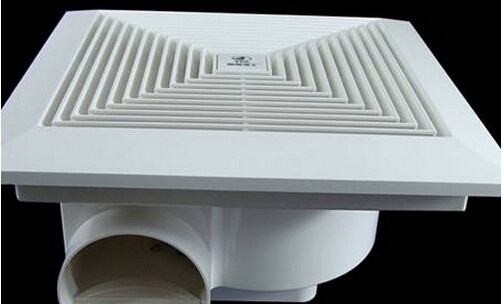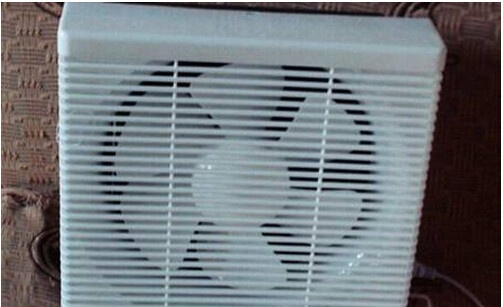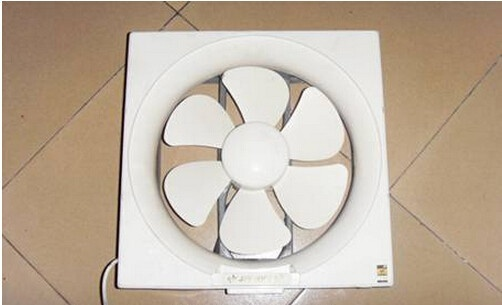|

# 厨卫通风换气好帮手 支招排风扇选购与安装

排气扇可以实现排气换气，又经济实惠。下文我们就介绍排气扇的选购与安装知识。Part1：排烟通风透气 家用排风扇介绍

农历新年就在眼前，相信厨房将成为很多人家最热闹的空间。不过想想如果这时候油烟不能及时散去，十分呛人，相信会影响到过年的心情甚至身体健康。卫浴也是如此，洗澡时水汽不能及时散去也是一件烦人的事。而安装排风扇，将在很大程度上避免此类问题出现。

一、排风扇介绍

又称通风扇，是由电动机带动风叶旋转驱动气流，促使室内外空气交换的一类空气调节电器。排风扇的目的就是除去室内的污浊空气，调节温度、湿度，维持室内空气正常情况。

二、排风扇的种类

1、按换气方式分

排风扇按照换气方式的不同，可分为排出式、吸入式以及并用式三种。顾名思义，排出式就是只负责排出室内污浊空气，由其他进风口负责进入新鲜空气;而吸入式和排出式正好相反，其只负责吸入新鲜空气，由其他通道排出污浊空气;并用式则是结合两种，室内吸气与排气都有其来负责。

我们提醒：业主在选择排风扇时，需要根据厨卫等安装空间的情况来选择合适换气方式的产品。比如如果厨卫面积较小，而且没有窗户等，那么建议选用并用式。

2、按进排气口分

排风扇还可按照进排气口分为隔墙型、管道排气型、管道进气型以及全管道型。隔墙型是指安装在墙面上，安装墙体的两侧都是自由空间，可直接通过隔墙的一侧向另一侧换气;而管道排气型则是一侧从自由空间进气，另一侧通过导管排气;管道进气型与管道排气型正好相反;此外还有全管道型，则是指排气扇两侧都安装管道，通过管道进气与排气。

我们提醒：业主在选择排风扇时，除了考虑产品的换气方式，还需要根据安装空间的情况以及安装位置选择合适的产品型号，比如是带有管道的还是不需要导管的。

3、按安装位置分

按照排风扇的安装位置，又可分为壁挂式、窗口式、天花板式几种。

壁挂式排风扇：排风扇直接安装在墙壁的孔洞上，壁挂式排风扇一般体积较小，由于其横截面较窄，导致换气力度较弱，一般适合比较小而封闭的空间使用。

窗口式排风扇：将排风扇安装在窗户上，这样不仅不用在墙壁上开孔洞，而且节省了排气管道。但是窗口式排风扇占用了窗户面积，对光线也有阻挡作用，在选购时需注意到这点。

天花板式排风扇：排风扇安装在天花板上，较为美观。不过此种安装方式，通常需要配备适合长度的通风管道。Part2：综合考虑 厨卫排风扇选购支招

排气扇具有价低、风量大、耗能小等特点，是普通家庭室内通风换气的较佳选择，即使是有能力安装油烟机等产品的家庭，安装排风扇作为辅助换气装置也是不错的选择。关于排风扇应该如何选购，相信通过前面了解了排风扇种类的业主心中应该有一定把握了。下面再给大家支点招，确保都能买到适合自家的排风扇产品。

一、厨房排风扇选购

厨房是烹饪美食的地方，往往油烟会成为我们头疼的问题。在选用厨房用的排风扇时，除了根据空间选择合适换气方式等的产品外，还需要重点注意排风扇的功率。

厨房宜选大功率的排风扇

厨房的油烟需要瞬时快速排出，因此相比于卫浴间，厨房要选择功率较大的排风扇产品。尽量选择较大功率和叶轮尺寸的免清洗排风扇，必要时可安装2—3台排气扇。此外，注意不要选天花板式排风扇，毕竟厨房油烟较多，安装在顶上不仅排烟效果一般，且清洁不易。

二、卫浴间排风扇选购

市场上的家用排气扇分吸顶式、壁挂式和窗式三种。卫生间是潮气、湿气的集中区域，选购的时候应根据卫生间的面积大小，通风环境来考虑，还要确定安装在吊顶上还是安装在墙上或窗户上，根据不同的安装位置，选购不同型号的排风扇。

三、排风扇选购支招

1、注意排风扇的噪音

在选购排风扇时，还要留意其响声的等级，等级越低的排风扇越好，通常小于三级的是可以接受。

2、检查外观

优质的排风扇无论从用料和做工方面都是比较好的，不会出现用料单薄、接缝不均、变形破损的现象。选购时查看排风扇平面是否无翘曲现象，塑料件平整光滑，电镀件光亮，没有锈斑痕迹。

3、试机检查

选购时，最好将排风扇现场试下。通电后观察排风扇的风叶是否旋转平稳、无异常杂声与振动，风量足够等。Part3：注重细节 厨卫排风扇安装需知

前面我们已经了解到排风扇按照安装位置的不同可分为几种类型。下面我们就分别来看不同类型排风扇的安装方法，以及厨卫等排风扇的清洁养护知识。

一、挂墙式排风扇安装

当排风扇安装在墙壁上时，要先量准排气扇轮廓尺寸和螺丝孔安装的确切位置，然后用钢凿或手提式冲击钻在墙体上凿出安装孔。然后在洞孔内埋置木块(或木枕)，用砂浆嵌实，等砂浆嵌实后即可安装排气扇。

二、窗口式排风扇安装

当要将排风扇安装在窗户上时，一般流程是，首先卸去一块玻璃，用电钻在窗框上钻孔后再钻螺纹，用螺丝固定。如窗框大于排气扇，可预先备一块白铁皮拧上，再将排气扇固定在铁皮上，铁皮上可涂油漆，固定方式为对拧螺丝或铆钉。

三、天花板式排风扇安装

如果吊顶式铝扣板吊顶，一般都会配有与吊顶龙骨配套的金属扣件，安装非常方便;而如果是木龙骨吊顶，只要接通排风管，用木螺丝拧紧排风扇面板就行。四、排风扇安装需知

1、安装前检查

排风扇安装前应仔细检查风机是否完整无损，各紧固件螺栓是否有松动或脱落，叶轮有无碰撞风罩。认真检查扇叶或百叶在运输过程中有无变形受损。

2、安装要点

排风扇安装需平稳，风机安装时注意风机的水平位置，不可有倾斜现象。风机安装完成后，要对其周围密封性进行检查。如有空隙，可用阳光板或者玻璃胶进行密封。

3、安装验收

排风扇安装完成后，需检查风机内部是否留有遗留的工具和杂物。用手或杠杆拨动扇叶，检查是否有过紧或擦碰现象，有无妨碍转动的物品无异常现象，方可进行试运转;运转中如出现风机振动或电机有“ 嗡嗡 ”的异常声响或其它异常现象应停机检查并修复。

`声明：本文由入驻焦点开放平台的作者撰写，除焦点官方账号外，观点仅代表作者本人，不代表焦点立场错误信息举报电话： 400-099-0099，邮箱：jubao@vip.sohu.com，或点此进行意见反馈，或点此进行举报投诉。`A B C D E F G H J K L M N P Q R S T W X Y Z
A - B - C - D - E
• A
• 鞍山
• 安庆
• 安阳
• 安顺
• 安康
• 澳门
• B
• 北京
• 保定
• 包头
• 巴彦淖尔
• 本溪
• 蚌埠
• 亳州
• 滨州
• 北海
• 百色
• 巴中
• 毕节
• 保山
• 宝鸡
• 白银
• 巴州
• C
• 承德
• 沧州
• 长治
• 赤峰
• 朝阳
• 长春
• 常州
• 滁州
• 池州
• 长沙
• 常德
• 郴州
• 潮州
• 崇左
• 重庆
• 成都
• 楚雄
• 昌都
• 慈溪
• 常熟
• D
• 大同
• 大连
• 丹东
• 大庆
• 东营
• 德州
• 东莞
• 德阳
• 达州
• 大理
• 德宏
• 定西
• 儋州
• 东平
• E
• 鄂尔多斯
• 鄂州
• 恩施
F - G - H - I - J
• F
• 抚顺
• 阜新
• 阜阳
• 福州
• 抚州
• 佛山
• 防城港
• G
• 赣州
• 广州
• 桂林
• 贵港
• 广元
• 广安
• 贵阳
• 固原
• H
• 邯郸
• 衡水
• 呼和浩特
• 呼伦贝尔
• 葫芦岛
• 哈尔滨
• 黑河
• 淮安
• 杭州
• 湖州
• 合肥
• 淮南
• 淮北
• 黄山
• 菏泽
• 鹤壁
• 黄石
• 黄冈
• 衡阳
• 怀化
• 惠州
• 河源
• 贺州
• 河池
• 海口
• 红河
• 汉中
• 海东
• 怀来
• I
• J
• 晋中
• 锦州
• 吉林
• 鸡西
• 佳木斯
• 嘉兴
• 金华
• 景德镇
• 九江
• 吉安
• 济南
• 济宁
• 焦作
• 荆门
• 荆州
• 江门
• 揭阳
• 金昌
• 酒泉
• 嘉峪关
K - L - M - N - P
• K
• 开封
• 昆明
• 昆山
• L
• 廊坊
• 临汾
• 辽阳
• 连云港
• 丽水
• 六安
• 龙岩
• 莱芜
• 临沂
• 聊城
• 洛阳
• 漯河
• 娄底
• 柳州
• 来宾
• 泸州
• 乐山
• 六盘水
• 丽江
• 临沧
• 拉萨
• 林芝
• 兰州
• 陇南
• M
• 牡丹江
• 马鞍山
• 茂名
• 梅州
• 绵阳
• 眉山
• N
• 南京
• 南通
• 宁波
• 南平
• 宁德
• 南昌
• 南阳
• 南宁
• 内江
• 南充
• P
• 盘锦
• 莆田
• 平顶山
• 濮阳
• 攀枝花
• 普洱
• 平凉
Q - R - S - T - W
• Q
• 秦皇岛
• 齐齐哈尔
• 衢州
• 泉州
• 青岛
• 清远
• 钦州
• 黔南
• 曲靖
• 庆阳
• R
• 日照
• 日喀则
• S
• 石家庄
• 沈阳
• 双鸭山
• 绥化
• 上海
• 苏州
• 宿迁
• 绍兴
• 宿州
• 三明
• 上饶
• 三门峡
• 商丘
• 十堰
• 随州
• 邵阳
• 韶关
• 深圳
• 汕头
• 汕尾
• 三亚
• 三沙
• 遂宁
• 山南
• 商洛
• 石嘴山
• T
• 天津
• 唐山
• 太原
• 通辽
• 铁岭
• 泰州
• 台州
• 铜陵
• 泰安
• 铜仁
• 铜川
• 天水
• 天门
• W
• 乌海
• 乌兰察布
• 无锡
• 温州
• 芜湖
• 潍坊
• 威海
• 武汉
• 梧州
• 渭南
• 武威
• 吴忠
• 乌鲁木齐
X - Y - Z
• X
• 邢台
• 徐州
• 宣城
• 厦门
• 新乡
• 许昌
• 信阳
• 襄阳
• 孝感
• 咸宁
• 湘潭
• 湘西
• 西双版纳
• 西安
• 咸阳
• 西宁
• 仙桃
• 西昌
• Y
• 运城
• 营口
• 盐城
• 扬州
• 鹰潭
• 宜春
• 烟台
• 宜昌
• 岳阳
• 益阳
• 永州
• 阳江
• 云浮
• 玉林
• 宜宾
• 雅安
• 玉溪
• 延安
• 榆林
• 银川
• Z
• 张家口
• 镇江
• 舟山
• 漳州
• 淄博
• 枣庄
• 郑州
• 周口
• 驻马店
• 株洲
• 张家界
• 珠海
• 湛江
• 肇庆
• 中山
• 自贡
• 资阳
• 遵义
• 昭通
• 张掖
• 中卫

1室1厅1厨1卫1阳台

1
2
3
4
5

0
1
2

1

1

0
1
2
3报名成功，资料已提交审核A B C D E F G H J K L M N P Q R S T W X Y Z
A - B - C - D - E
• A
• 鞍山
• 安庆
• 安阳
• 安顺
• 安康
• 澳门
• B
• 北京
• 保定
• 包头
• 巴彦淖尔
• 本溪
• 蚌埠
• 亳州
• 滨州
• 北海
• 百色
• 巴中
• 毕节
• 保山
• 宝鸡
• 白银
• 巴州
• C
• 承德
• 沧州
• 长治
• 赤峰
• 朝阳
• 长春
• 常州
• 滁州
• 池州
• 长沙
• 常德
• 郴州
• 潮州
• 崇左
• 重庆
• 成都
• 楚雄
• 昌都
• 慈溪
• 常熟
• D
• 大同
• 大连
• 丹东
• 大庆
• 东营
• 德州
• 东莞
• 德阳
• 达州
• 大理
• 德宏
• 定西
• 儋州
• 东平
• E
• 鄂尔多斯
• 鄂州
• 恩施
F - G - H - I - J
• F
• 抚顺
• 阜新
• 阜阳
• 福州
• 抚州
• 佛山
• 防城港
• G
• 赣州
• 广州
• 桂林
• 贵港
• 广元
• 广安
• 贵阳
• 固原
• H
• 邯郸
• 衡水
• 呼和浩特
• 呼伦贝尔
• 葫芦岛
• 哈尔滨
• 黑河
• 淮安
• 杭州
• 湖州
• 合肥
• 淮南
• 淮北
• 黄山
• 菏泽
• 鹤壁
• 黄石
• 黄冈
• 衡阳
• 怀化
• 惠州
• 河源
• 贺州
• 河池
• 海口
• 红河
• 汉中
• 海东
• 怀来
• I
• J
• 晋中
• 锦州
• 吉林
• 鸡西
• 佳木斯
• 嘉兴
• 金华
• 景德镇
• 九江
• 吉安
• 济南
• 济宁
• 焦作
• 荆门
• 荆州
• 江门
• 揭阳
• 金昌
• 酒泉
• 嘉峪关
K - L - M - N - P
• K
• 开封
• 昆明
• 昆山
• L
• 廊坊
• 临汾
• 辽阳
• 连云港
• 丽水
• 六安
• 龙岩
• 莱芜
• 临沂
• 聊城
• 洛阳
• 漯河
• 娄底
• 柳州
• 来宾
• 泸州
• 乐山
• 六盘水
• 丽江
• 临沧
• 拉萨
• 林芝
• 兰州
• 陇南
• M
• 牡丹江
• 马鞍山
• 茂名
• 梅州
• 绵阳
• 眉山
• N
• 南京
• 南通
• 宁波
• 南平
• 宁德
• 南昌
• 南阳
• 南宁
• 内江
• 南充
• P
• 盘锦
• 莆田
• 平顶山
• 濮阳
• 攀枝花
• 普洱
• 平凉
Q - R - S - T - W
• Q
• 秦皇岛
• 齐齐哈尔
• 衢州
• 泉州
• 青岛
• 清远
• 钦州
• 黔南
• 曲靖
• 庆阳
• R
• 日照
• 日喀则
• S
• 石家庄
• 沈阳
• 双鸭山
• 绥化
• 上海
• 苏州
• 宿迁
• 绍兴
• 宿州
• 三明
• 上饶
• 三门峡
• 商丘
• 十堰
• 随州
• 邵阳
• 韶关
• 深圳
• 汕头
• 汕尾
• 三亚
• 三沙
• 遂宁
• 山南
• 商洛
• 石嘴山
• T
• 天津
• 唐山
• 太原
• 通辽
• 铁岭
• 泰州
• 台州
• 铜陵
• 泰安
• 铜仁
• 铜川
• 天水
• 天门
• W
• 乌海
• 乌兰察布
• 无锡
• 温州
• 芜湖
• 潍坊
• 威海
• 武汉
• 梧州
• 渭南
• 武威
• 吴忠
• 乌鲁木齐
X - Y - Z
• X
• 邢台
• 徐州
• 宣城
• 厦门
• 新乡
• 许昌
• 信阳
• 襄阳
• 孝感
• 咸宁
• 湘潭
• 湘西
• 西双版纳
• 西安
• 咸阳
• 西宁
• 仙桃
• 西昌
• Y
• 运城
• 营口
• 盐城
• 扬州
• 鹰潭
• 宜春
• 烟台
• 宜昌
• 岳阳
• 益阳
• 永州
• 阳江
• 云浮
• 玉林
• 宜宾
• 雅安
• 玉溪
• 延安
• 榆林
• 银川
• Z
• 张家口
• 镇江
• 舟山
• 漳州
• 淄博
• 枣庄
• 郑州
• 周口
• 驻马店
• 株洲
• 张家界
• 珠海
• 湛江
• 肇庆
• 中山
• 自贡
• 资阳
• 遵义
• 昭通
• 张掖
• 中卫• 手机• 分享
• 设计
免费设计
• 计算器
装修计算器
• 入驻
合作入驻
• 联系
联系我们
• 置顶
返回顶部